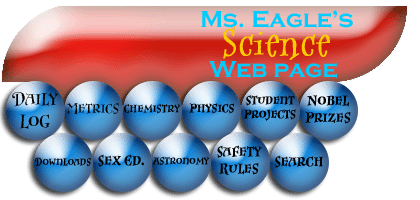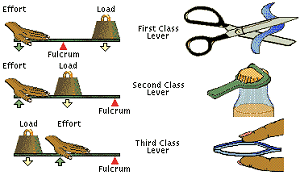# Work, Power, and Simple MachinesWork- The product of the force applied to an object and the distance the object moves. The unit of work is the joule (J). The formula for work is Force x Distance.

Power- The amount of work done per unit of time. The unit for power, joules/second, is the watt (w).

Simple Machine- Makes work easier to do.

Lever- A simple machine that involves two forces and a pivot point called a fulcrum.

Mechanical Advantage(MA)- A way to express the benefit of a machine. A number without units. If the MA is more than 1, the machine makes work easier by multiplying the effort force. If the MA is less than 1, the machine makes work easier by allowing the resistance to move farther and faster than the effort. If the MA is exactly 1, the machine makes work easier by changing the direction in which the effort must be applied.

Fulcrum- Support on which a lever turns.